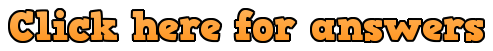# Surface Area of a Sphere Textbook AnswersWorkout

Question 1:

(a)   314.16cm²

(b)   2463.01cm²

(c)   113.10cm²

(d)   21.24cm²

(e)  2290.22cm²

(f)  2.54mm²

Question 2:

(a)  196𝛑 cm²

(b)  64𝛑 cm²

(c)  4.84𝛑 m²

Question 3:

(a)   12.6cm²

(b)   16300mm²

(c)   2.01m²

(d)   13.5 square inches

Question 4:

(a)   1.99cm

(b)   8.65cm

(c)   39.09cm

Question 5:

(a)  2cm

(b)  5cm

(c)  60cm

Apply

Question 1:   190.852cm²

Question 2:   3cm radius – SA = 113.1cm² and 6cm radius – SA = 452.4cm², therefore 4 times larger

Question 3:  r = √(A/4pi)

Question 4:  Peter is incorrect

Approach 1: SA of Cube: 6x²  and SA sphere is 4𝛑x².

4𝛑 is approx 12.566, which is more than twice 6.

Approach 2: let x = 10cm (for example)

Sphere 1256.6cm² and cube 600cm²

So the surface area of the sphere is more than twice the cube’s

Question 6:

4𝛑x² = 2𝛑x² + 2𝛑xh

2𝛑x² = 2𝛑xh

x² = xh

x = h (since x ≠ 0)